##### Question

In: Statistics and Probability

# An enginner is going to redesign  an ejection seat for airplane. the seat was designed for pilots...

An enginner is going to redesign  an ejection seat for airplane. the seat was designed for pilots weighing between 150 Ib and 201 Ib . the new population of pilots has normally distributed weights with a mean of 157 Ib and a standard deviation of 29.7 Ib.

b) if 38 different pilots are randomly selected, find the probability that their mean weight is between 150 Ib and 201 Ib.

the probability is approximately ___

## Solutions

##### Expert Solution

Solution :

Given that ,

mean =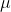= 157

standard deviation =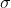= 29.7

P( 150 < x < 201 )

= P[( 150 - 157 ) / 29.7 ) < (x -) /< ( 201 - 157 ) / 29.7 ) ]

= P( -0.24 < z < 1.48 )

= P(z < 1.48 ) - P(z < -0.24 )

Using z table,

= 0.9306 - 0.4052

= 0.5254

Probability = 0.5254

( b )

n = 38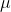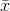= 157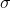=/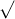n = 29.7 /38 = 4.8180

P( 150 << 201 )

= P[( 150 - 157 ) / 4.8180 < (-) /< ( 201 - 157 ) / 4.8180 )]

= P( -1.45 < Z < 9.13 )

= P(Z < 9.13 ) - P(Z < -1.45 )

Using z table,

= 1.0000 - 0.0735

= 0.9265

Probability = 0.9265

## Related Solutions

##### An engineer is going to redesign an ejection seat for an airplane. The seat was designed...
An engineer is going to redesign an ejection seat for an airplane. The seat was designed for pilots weighing between 150 lb and 201 lb. The new population of pilots has normally distributed weights with a mean of 159 lb and a standard deviation of 26.6 lb. A. If a pilot is randomly​ selected, find the probability that his weight is between 150 lb and 201 lb.The probability is approximately______? ​(Round to four decimal places as​ needed.) B. If 40...
##### An engineer is going to redesign an ejection seat for an airplane. The seat was designed...
An engineer is going to redesign an ejection seat for an airplane. The seat was designed for pilots weighing between 130 lb and 171 lb. The new population of pilots has normally distributed weights with a mean of 138 lb and a standard deviation of 34.8 lb. a. If a pilot is randomly​ selected, find the probability that his weight is between 130 lb and 171 lb. The probability is approximately________. ​ (Round to four decimal places as​ needed.) b....
##### An engineer is going to redesign an ejection seat for an airplane. The seat was designed...
An engineer is going to redesign an ejection seat for an airplane. The seat was designed for pilots weighing between 120 lb and 171 lb. The new population of pilots has normally distributed weights with a mean of 130 lb and a standard deviation of 33.7lb. a. If a pilot is randomly selected, find the probability that his weight is between 120 lb and 171 lb. The probability is approximately __. (Round to four decimal places as needed.) b. If...
##### An engineer is going to redesign an ejection seat for an airplane. The seat was designed...
An engineer is going to redesign an ejection seat for an airplane. The seat was designed for pilots weighing between 130 lb and 191 lb. The new population of pilots has normally distributed weights with a mean of 140 lb and a standard deviation of 26.2 lb. a. If a pilot is randomly​ selected, find the probability that his weight is between 130 lb and 191 lb. The probability is approximately nothing. ​(Round to four decimal places as​ needed.) b....
##### An engineer is going to redesign an ejection seat for an airplane. The seat was designed...
An engineer is going to redesign an ejection seat for an airplane. The seat was designed for pilots weighing between 130 lb and 171 lb. The new population of pilots has normally distributed weights with a mean of 137 lb and a standard deviation of 29.5 lb . A) If a pilot is randomly​ selected, find the probability that his weight is between 130lb and 171lb. ​(Round to four decimal places as​ needed.) B) If 37 different pilots are randomly​...
##### An engineer is going to redesign an ejection seat for an airplane. The seat was designed...
An engineer is going to redesign an ejection seat for an airplane. The seat was designed for pilots weighing between 120 lb and 161 lb. The new population of pilots has normally distributed weights with a mean of 129 lb and a standard deviation of 29.1 lb. a. If a pilot is randomly​ selected, find the probability that his weight is between 120 lb and 161 lb. The probability is approximately _____. ​(Round to four decimal places as​ needed.) b....
##### An engineer is going to redesign an ejection seat for an airplane. The seat was designed...
An engineer is going to redesign an ejection seat for an airplane. The seat was designed for pilots weighing between 150 lb and 191 lb. The new population of pilots has normally distributed weights with a mean of 156 lb and a standard deviation of 29.1 lb. A. If a pilot is randomly​ selected, find the probability that his weight is between 150 lb and 191 lb. The probability is approximately_______? ​(Round to four decimal places as​ needed.) B. If...
##### An engineer is going to redesign an ejection seat for an airplane. The seat was designed...
An engineer is going to redesign an ejection seat for an airplane. The seat was designed for pilots weighing between 140 lb and 181 lb. The new population of pilots has normally distributed weights with a mean of 149 lb and a standard deviation of 27.1 lb a. If a pilot is randomly​ selected, find the probability that his weight is between 140 lb and 181 lb. b. If 31 different pilots are randomly​ selected, find the probability that their...
##### An engineer is going to redesign an ejection seat for an airplane. The seat was designed...
An engineer is going to redesign an ejection seat for an airplane. The seat was designed for pilots weighing between 140 lb and 191 lb. The new population of pilots has normally distributed weights with a mean of 145 lb and a standard deviation of 30.1 lb. b. If 36 different pilots are randomly​ selected, find the probability that their mean weight is between 140 lb and 191 lb. The probability is approximately nothing. ​(Round to four decimal places as​...
##### An engineer is going to redesign an ejection seat for an airplane. The seat was designed...
An engineer is going to redesign an ejection seat for an airplane. The seat was designed for pilots weighing between 130 lb and 171 lb. The new population of pilots has normally distributed weights with a mean of 138 lb and a standard deviation of 31.6 lb. a. If a pilot is randomly​ selected, find the probability that his weight is between 130 lb and 171 lb. The probability is approximately ? ​(Round to four decimal places as​ needed.) b....